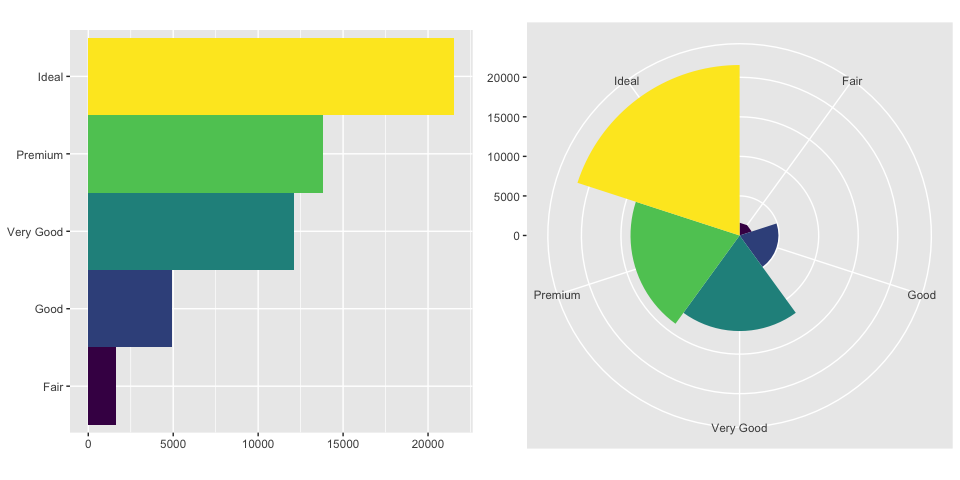# Position Adjustments & Coordinate Systems in ggplot2

## Position Adjustments

library(tidyverse)
ggplot(data = diamonds) +
geom_bar(mapping = aes(x = cut, fill = cut))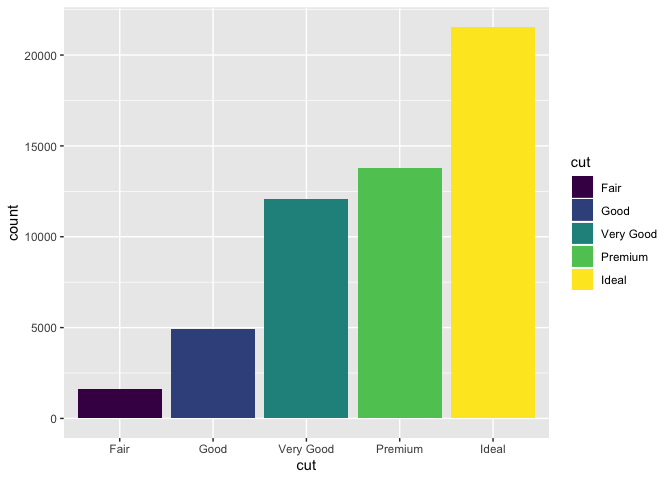• What happens if you map the fill aesthetic to another variable, like clarity: the bars are automatically stacked. Each colored rectangle represents a combination of cut and clarity.
p1.1 <- ggplot(data = diamonds) +
geom_bar(mapping = aes(x = cut, fill = clarity))
p1.1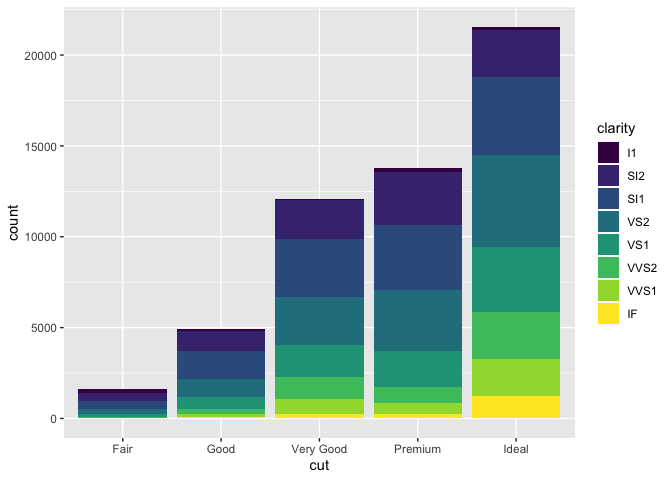• The stacking is performed automatically by the position adjustment specified by the position argument.
• For bar charts, position takes the following values: "stack", "identity","fill" or "dodge".
• The default value for position in geom_bar() is "stack".

### “identity”

• position = "identity" will place each object exactly where it falls in the context of the graph.
• The identity position adjustment is more useful for 2d geoms, like points, where it is the default.
library(gridExtra)
p1.2 <- ggplot(data = diamonds) +
geom_bar( mapping = aes(x = cut, fill = clarity), position = "identity")
grid.arrange(p1.1, p1.2, nrow = 1)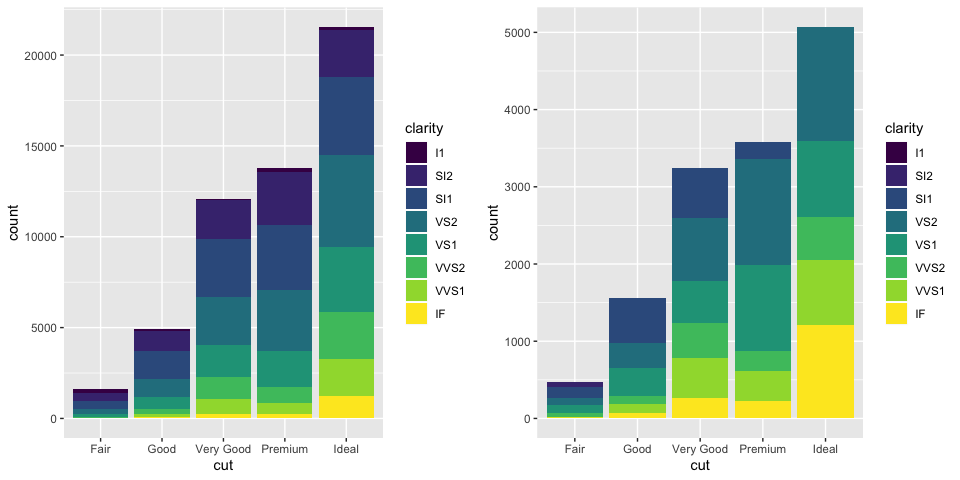• To see that overlapping we either need to make the bars slightly transparent by setting alpha to a small value, or completely transparent by setting fill = NA.
p2.1 <- ggplot(data = diamonds, mapping = aes(x = cut, fill = clarity)) +
geom_bar(alpha = 1/5, position = "identity")
p2.2 <- ggplot(data = diamonds, mapping = aes(x = cut, colour = clarity)) +
geom_bar(fill = NA, position = "identity")
grid.arrange(p2.1, p2.2, nrow = 1)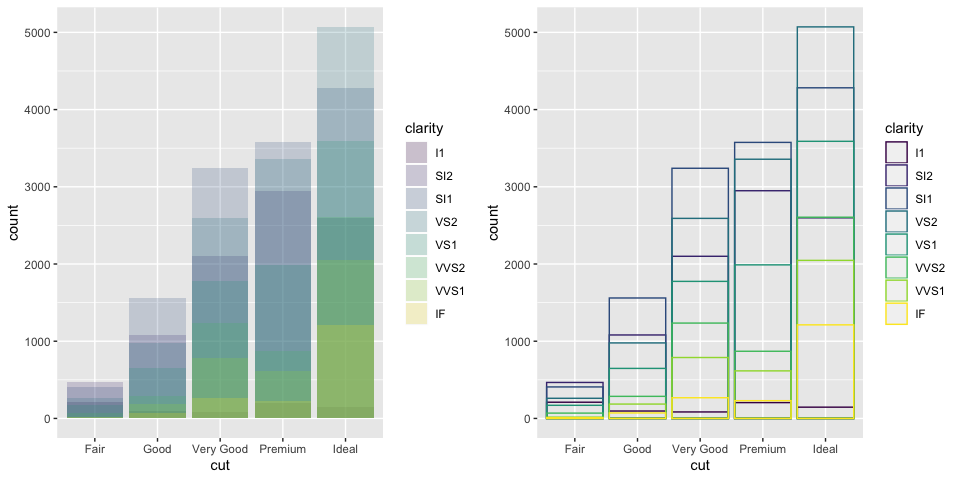### “fill”

• position = "fill" works like stacking, but makes each set of stacked bars the same height. This makes it easier to compare proportions across groups.
ggplot(data = diamonds) +
geom_bar(mapping = aes(x = cut, fill = clarity), position = "fill")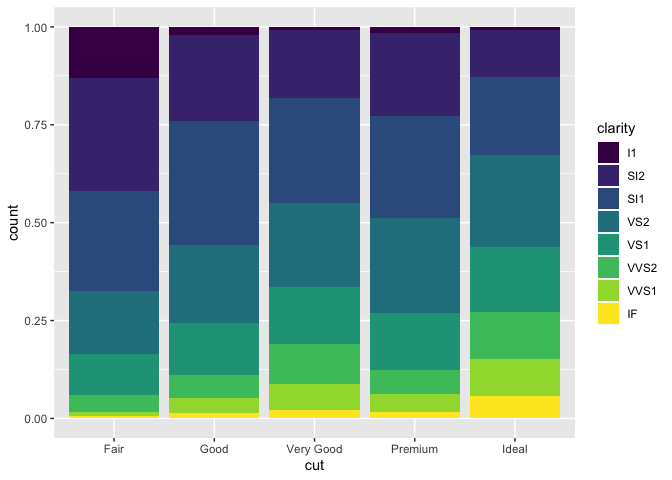### “dodge”

• position = "dodge" places overlapping objects directly beside one another. This makes it easier to compare individual values.
ggplot(data = diamonds) +
geom_bar(mapping = aes(x = cut, fill = clarity), position = "dodge")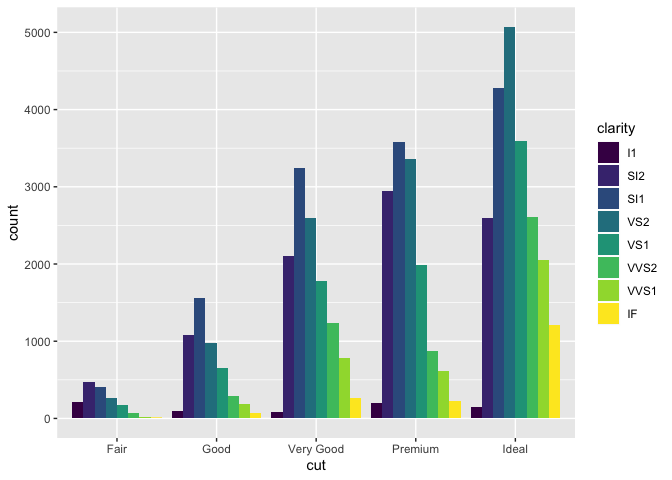• Notes: To learn more about a position adjustment, look up the help page associated with each adjustment: ?position_dodge, ?position_fill, ?position_identity, ?position_jitter, and ?position_stack.

### “jitter” in geom_point()

ggplot(data = mpg) +
geom_point(mapping = aes(x = displ, y = hwy))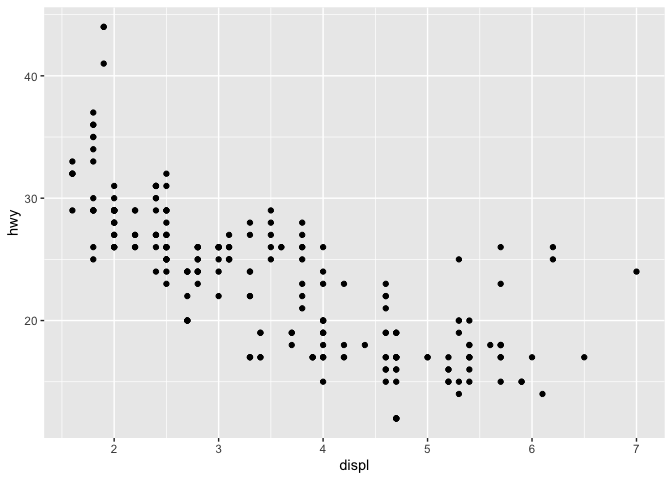• In this plot, there are only 126 points, even though there are 234 observations in the dataset.
• The values of hwy and displ are rounded so the points appear on a grid and many points overlap each other. This problem is known as overplotting.

• By setting position = “jitter”, R adds a small amount of random noise to each point. This spreads the points out because no two points are likely to receive the same amount of random noise.

ggplot(data = mpg) +
geom_point(mapping = aes(x = displ, y = hwy), position = "jitter")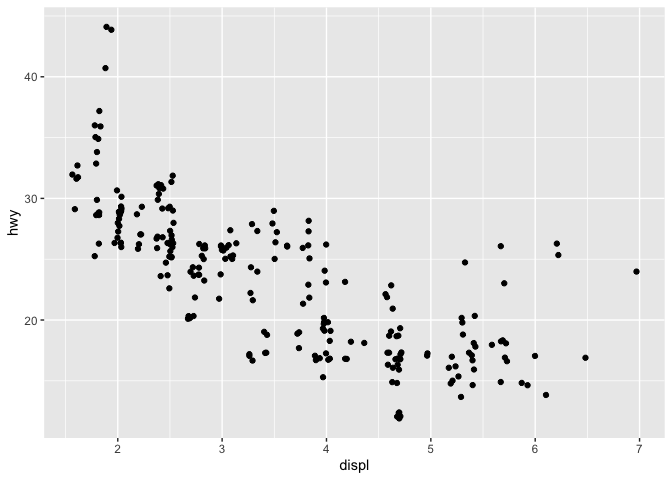• ggplot2 comes with a shorthand for geom_point(position = "jitter"): geom_jitter().

## Coordinate Systems

• The default coordinate system is the Cartesian coordinate system where the x and y positions act independently to determine the location of each point.

### coord_flip()

• coord_flip() switches the x and y axes. This is useful (for example), if you want horizontal boxplots.
p3.1 <- ggplot(data = mpg, mapping = aes(x = class, y = hwy)) +
geom_boxplot()

p3.2 <- ggplot(data = mpg, mapping = aes(x = class, y = hwy)) +
geom_boxplot() +
coord_flip()

grid.arrange(p3.1, p3.2, nrow = 1)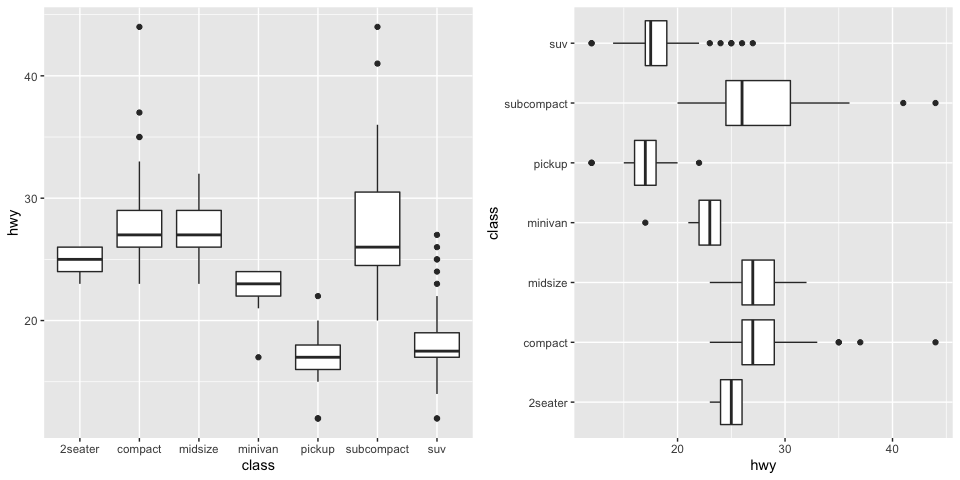### coord_quickmap()

• coord_quickmap() sets the aspect ratio correctly for maps. This is very important if you’re plotting spatial data with ggplot2.
• To run the following code, you first need to install the maps package.
library(maps)
nz <- map_data("nz")

p4.1 <- ggplot(nz, aes(long, lat, group = group)) +
geom_polygon(fill = "white", colour = "black")

p4.2 <- ggplot(nz, aes(long, lat, group = group)) +
geom_polygon(fill = "white", colour = "black") +
coord_quickmap()

grid.arrange(p4.1, p4.2, nrow = 1)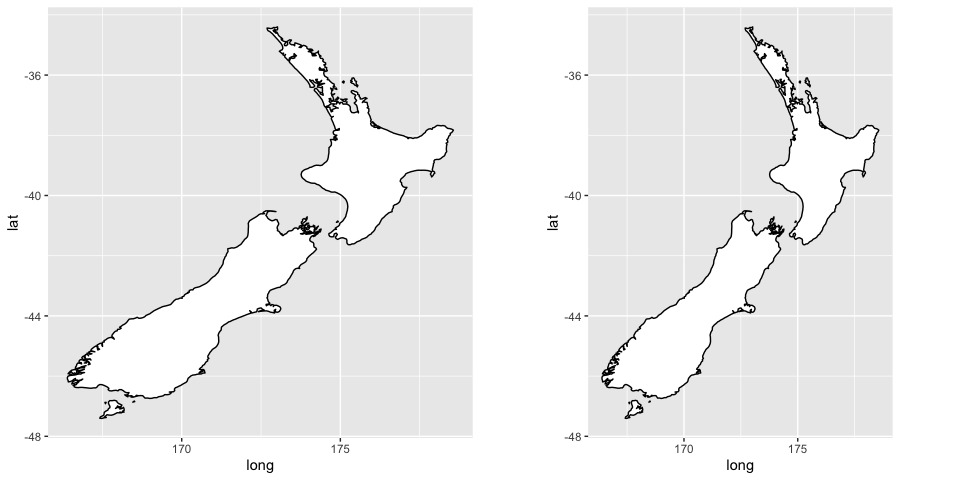### coord_polar()

• coord_polar() uses polar coordinates. Polar coordinates reveal an interesting connection between a bar chart and a Coxcomb chart.
bar <- ggplot(data = diamonds) +
geom_bar(
mapping = aes(x = cut, fill = cut),
show.legend = FALSE,
width = 1
) +
theme(aspect.ratio = 1) +
labs(x = NULL, y = NULL)

p5.1 <- bar + coord_flip()
p5.2 <- bar + coord_polar()

grid.arrange(p5.1, p5.2, nrow = 1)